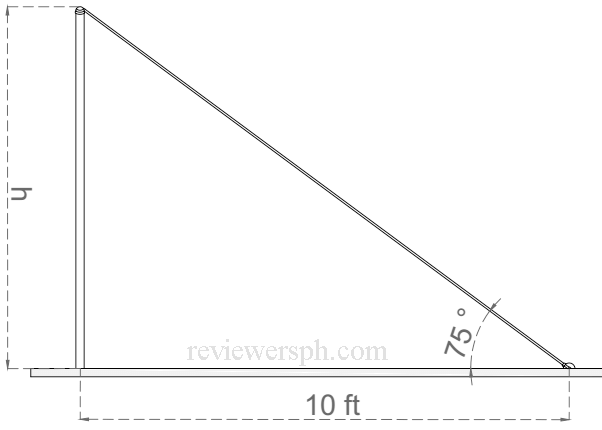### Math Notes

Subjects

#### Trigonometry Solutions

##### Topics || Problems

A wire is stretched from the top of a vertical pole standing on the level ground. The wire reaches to a point on the ground 10 feet from the foot of the pole and makes an angle of 75o with the horizontal. Find the height of the pole and the length of the wire.

Solution:$$\tan 75 = \frac{h}{10}$$

$$10 \tan 75 =h$$

$$h = 37.32 feet$$